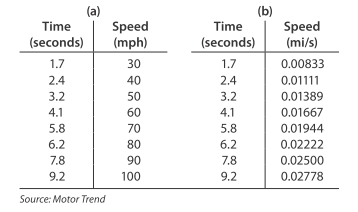# Modeling SRT Viper acceleration Table 13.1 (a) shows the time in seconds an SRT Viper requires to reach various speeds up to 100 mph. Table 13.1(b) shows the same data, hut with speeds in miles per second. Fit a power model to the data in Table 13.1 (b). Report the model with 3 significant digits. Use a definite integral from 0 to 9.2 of the function you found in part (a) to find the distance traveled by the Viper as it went from 0 mph to 100 mph in 9.2 seconds. Table 13.1### Mathematical Applications for the ...

11th Edition
Ronald J. Harshbarger + 1 other
Publisher: Cengage Learning
ISBN: 9781305108042

#### Solutions

Chapter
Section### Mathematical Applications for the ...

11th Edition
Ronald J. Harshbarger + 1 other
Publisher: Cengage Learning
ISBN: 9781305108042
Chapter 13.2, Problem 70E
Textbook Problem
1 views

## Modeling SRT Viper acceleration Table 13.1 (a) shows the time in seconds an SRT Viper requires to reach various speeds up to 100 mph. Table 13.1(b) shows the same data, hut with speeds in miles per second. Fit a power model to the data in Table 13.1 (b). Report the model with 3 significant digits. Use a definite integral from 0 to 9.2 of the function you found in part (a) to find the distance traveled by the Viper as it went from 0 mph to 100 mph in 9.2 seconds. Table 13.1This textbook solution is under construction.

### Still sussing out bartleby?

Check out a sample textbook solution.

See a sample solution

#### The Solution to Your Study Problems

Bartleby provides explanations to thousands of textbook problems written by our experts, many with advanced degrees!

Get Started